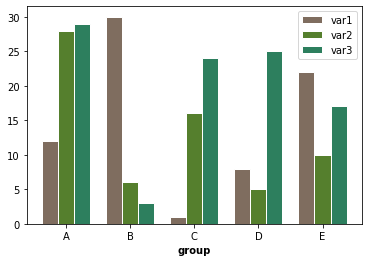You can plot a grouped barplot using the `bar()` function of matplotlib. The following example displays 5 different groups with their 3 variables. In order to do that, the values and positions of variables are passed to 3 `bar()` functions.

Note that if you want to turn the graph into a stacked area barplot, you can check the following post.

``````# libraries
import numpy as np
import matplotlib.pyplot as plt

# set width of bars
barWidth = 0.25

# set heights of bars
bars1 = [12, 30, 1, 8, 22]
bars2 = [28, 6, 16, 5, 10]
bars3 = [29, 3, 24, 25, 17]

# Set position of bar on X axis
r1 = np.arange(len(bars1))
r2 = [x + barWidth for x in r1]
r3 = [x + barWidth for x in r2]

# Make the plot
plt.bar(r1, bars1, color='#7f6d5f', width=barWidth, edgecolor='white', label='var1')
plt.bar(r2, bars2, color='#557f2d', width=barWidth, edgecolor='white', label='var2')
plt.bar(r3, bars3, color='#2d7f5e', width=barWidth, edgecolor='white', label='var3')

# Add xticks on the middle of the group bars
plt.xlabel('group', fontweight='bold')
plt.xticks([r + barWidth for r in range(len(bars1))], ['A', 'B', 'C', 'D', 'E'])

# Create legend & Show graphic
plt.legend()
plt.show()``````## Contact & Edit

👋 This document is a work by Yan Holtz. You can contribute on github, send me a feedback on twitter or subscribe to the newsletter to know when new examples are published! 🔥